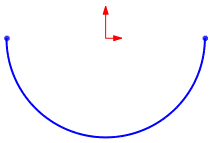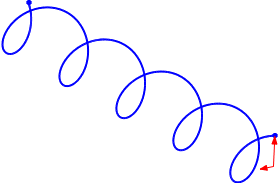# Equation Driven Curves

When you create equation driven curves, the values you use must be in radians.

You cannot use global variables directly for equation driven curves. However, you can create a global variable and associate it with a dimension, then use the dimension in the equation for the curve.

 yx = sqrt( 4 - x ^ 2) x1 = -2 x2 = 2xt = 50*cos(t) yt = 50*sin(t) t1 = 0 t2 = pixt = 2*sin(t) yt = 2*cos(t) zt = t t1 = 0 t2 = 30